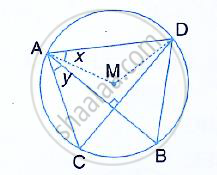Share

# In the Given Figure, M is the Centre of the Circle. Chords Ab and Cd Are Perpendicular to Each Other. If ∠Mad = X and ∠Bac = Y: - Mathematics

Course

#### Question

In the given figure, M is the centre of the circle. Chords AB and CD are perpendicular to each other.

If ∠MAD = x and ∠BAC = y :  express ∠AMD in terms of x.#### Solution

In the figure, M is the centre of the circle.
Chords AB and CD are perpendicular to each other at L.
∠MAD = x and ∠BAC = y

In  ∆AMD,

MA = MD

∴ ∠MAD = ∠MDA = x

But in ∆AMD,

∠MAD + ∠MDA + ∠AMD = 180°

⇒ x + x + ∠AMD = 180°

⇒ 2x + ∠AMD = 180°

⇒ ∠AMD =180° - 2x

Is there an error in this question or solution?